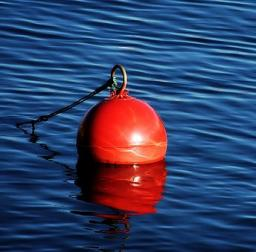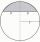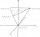# Float boya

A 0.5 meter spherical float is used as a location mark for a fishing boat anchor. It floats in salt water. Find the depth to which the float sinks if the material of which the float is made weighs 8 kilograms per cubic meter and salt water weighs 1027 kg/m3.

h =  0.0446 m

### Step-by-step explanation:Did you find an error or inaccuracy? Feel free to write us. Thank you!Tips to related online calculators
Looking for a statistical calculator?
Do you have a linear equation or system of equations and looking for its solution? Or do you have a quadratic equation?
Tip: Our Density units converter will help you with the conversion of density units.
Pythagorean theorem is the base for the right triangle calculator.

#### You need to know the following knowledge to solve this word math problem:

We encourage you to watch this tutorial video on this math problem:

## Related math problems and questions:

• Spherical capFrom the sphere of radius 11 was truncated spherical cap. Its height is 6. What part of the volume is a spherical cap from the whole sphere?
• Hollow sphereThe steel hollow sphere floats on the water plunged into half its volume. Determine the outer radius of the sphere and wall thickness, if you know that the weight of the sphere is 0.5 kg and the density of steel is 7850 kg/m3
• Spherical cap 4What is the surface area of a spherical cap, the base diameter 20 m, height 2.5 m? Calculate using formula.
• Sea waterMixing 62 kg of sea water with 84 kg rainwater is created water containing 3.1% salt. How many percent sea water contains salt?
• Centroid - two bodiesA body is composed of a 0.8 m long bar and a sphere with a radius of 0.1m attached so that its center lies on the longitudinal axis of the bar. Both bodies are of the same uniform material. The sphere is twice as heavy as the bar. Find the center of gravi
• Spherical capPlace a part of the sphere on a 4.6 cm cylinder so that the surface of this section is 20 cm2. Determine the radius r of the sphere from which the spherical cap was cut.
• Cork and swimmingIf a person weighs 80 kg, how many kilograms of cork must take a swimming belt to float on water? The density of the human body is 1050kg/m3 and cork 300kg/m3. (Instructions: Let the human body and cork on a mixture with a density of 1000kg/m3. When you m
• Spherical capThe spherical cap has a base radius of 8 cm and a height of 5 cm. Calculate the radius of a sphere of which this spherical cap is cut.
• Sphere floatingWill float a hollow iron ball with an outer diameter d1 = 20cm and an inside diameter d2 = 19cm in the water? The iron density is 7.8 g/cm3. (Instructions: Calculate the average sphere density and compare it with the water density. )
• Eiffel TowerEiffel Tower in Paris is 300 meters high and is made of steel. Its weight is 8000 tons. How tall is the tower model made ​​of the same material if it weighs is 2.4 kg?
• Center of gravityThe mass points are distributed in space as follows - specify by coordinates and weight. Find the center of gravity of the mass points system: A1 [1; -20; 3] m1 = 46 kg A2 [-20; 2; 9] m2 = 81 kg A3 [9
• LidWhat is the weight of concrete cover (lid) to round shape well with a diameter 1.8 m, if the thickness of the cover is 11 cm? 1 m3 of concrete weighs 2190 kg.
• The prison ballCalculate the density of the material that the prison ball is made from if you know its diameter is 15cm and its weight is approximately 2.3kg. With the help of mathematical-physicochemical tables estimate what material the ball is made from.
• Sphere - partsCalculate the area of a spherical cap, which is part of an area with base radius ρ = 9 cm and a height v = 3.1 cm.
• Spherical capWhat is the surface area of a spherical cap, the base diameter 20 m, height 2 m.
• The water tankThe water tank has the shape of a sphere with a radius of 2 m. How many liters of water will fit in the tank? How many kilograms of paint do we need to paint the tank, if we paint with 1 kg of paint 10 m2?
• CoG centerFind the position of the center of gravity of a system of four mass points having masses, m1, m2 = 2 m1, m3 = 3 m1, and m4 = 4 m1, if they lie at the vertices of an isosceles tetrahedron. (in all cases, between adjacent material points, the distance